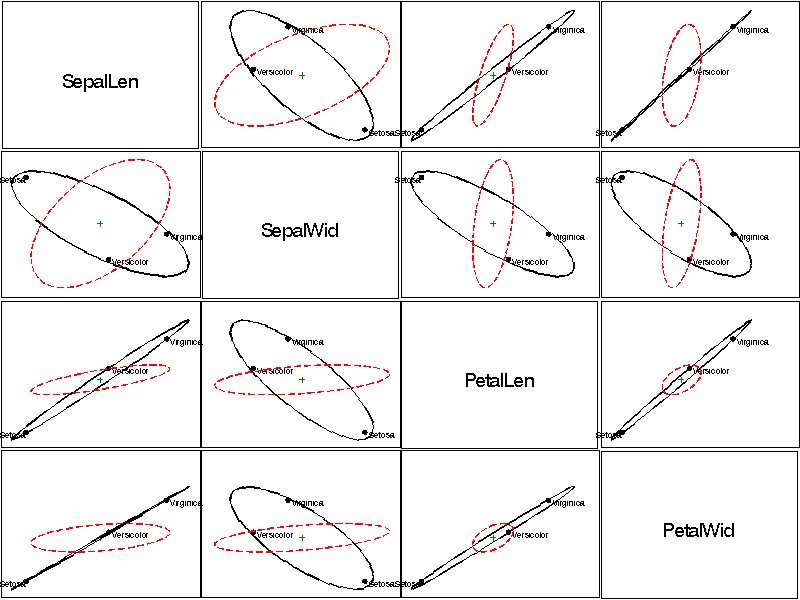hemat HE plots for all pairs of response variables hemat

# SAS Macro Programs: hemat

\$Version: 1.2-1 (25 Jan 2008)
Michael Friendly
York University

## The hemat macro (get hemat.sas)

### HE plots for all pairs of response variables

The HEMAT macro plots the covariance ellipses for a hypothesized (H) effect and for error (E) for all pairs of variables from a MANOVA or multivariate multiple regression.

## Usage

hemat is a macro program. Values must be supplied

The arguments may be listed within parentheses in any order, separated by commas. For example:

```  %hemat(data=iris, stat=stats,
var=SepalLen SepalWid PetalLen PetalWid,
effect=species);
```

### Parameters

Default values are shown after the name of each parameter.
DATA=
Name of the raw data set to be plotted [Default: `DATA=_LAST_`]
STAT=
Name of `OUTSTAT=` dataset from PROC GLM
EFFECT=
Name of MODEL effect to be displayed for the H matrix. This must be one of the terms on the right hand side of the MODEL statement used in the PROC GLM or PROC REG step, in the same format that this efffect is labeled in the `STAT=` dataset. This must be one of the values of the _SOURCE_ variable contained in the `STAT=` dataset.
VAR=
Names of response variables to be plotted - can be a list or X1-X4 or VARA--VARB [Default: `VAR=_NUMERIC_`]
NAMES=
Alternative variable names (used to label the diagonal cells.)
M1=
First matrix: either H or H+E [Default: M1=H]
M2=
Second matrix either E or I [Default: M2=E]
SCALE=
Scale factors for M1 and M2. See description in HEPLOT
HTITLE=
Height of variable name in diagonal cells
SYMBOLS=
Not used
COLORS=
Colors for the H and E ellipses [Default: `COLORS=BLACK RED`]
ANNO=
Annotate diag or off-diag plot [Default: `ANNO=NONE`]
GTEMP=
Temporary graphics catalog [Default: `GTEMP=GTEMP`]
KILL=
Delete grtemp when done [Default: `KILL=Y`]
GOUT=
Name of the graphic catalog [Default: `GOUT=GSEG`]

### Example

Determine how the variables in the Iris data set vary with species:
```%include macros(hemat);        *-- or include in an autocall library;
%include data(iris);

proc glm data=iris outstat=stats;
class species;
model SepalLen SepalWid PetalLen PetalWid = species / nouni ss3;
manova h=species;
run;

%hemat(data=iris, stat=stats,
var=SepalLen SepalWid PetalLen PetalWid,
effect=species
);
```
Output:### See also

biplot Generalized biplot of observations and variables
canplot Canonical discriminant structure plot
ellipses Plot bivariate data ellipses
hemreg Extract H and E matrices for multivariate regression
heplot Plot Hypothesis and Error matrices for a bivariate MANOVA effect
meanplot Plot means for factorial designs
panels Display a set of plots in rectangular panels